MTH4140 Home

# MTH 4140: Midterm 1 Practice 2

Problem 1

• (a) Provide an example of a simple graph that has $$5$$ vertices and $$7$$ edges;

• (b) Determine the complement of the graph you created in part (a) of the problem.

Problem 2

Which of the pictures below can be drawn without lifting the pen from the paper and without tracing the same line twice?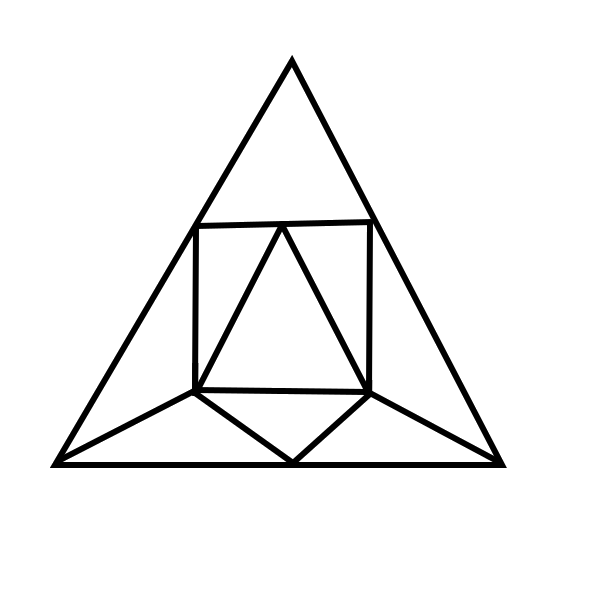$$\quad$$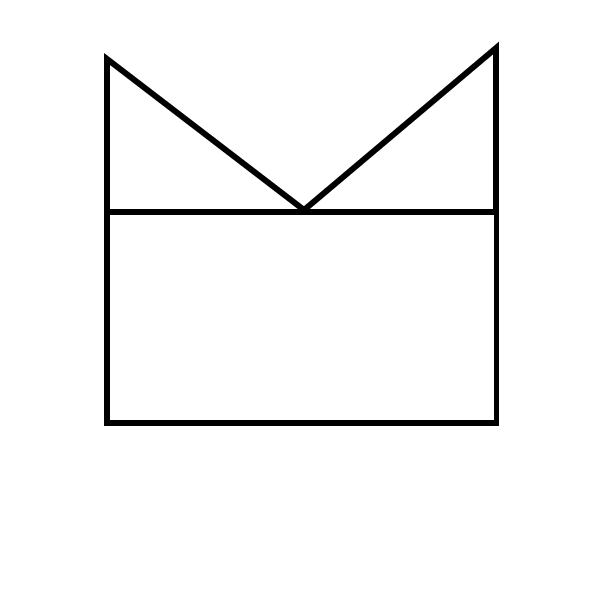$$\quad$$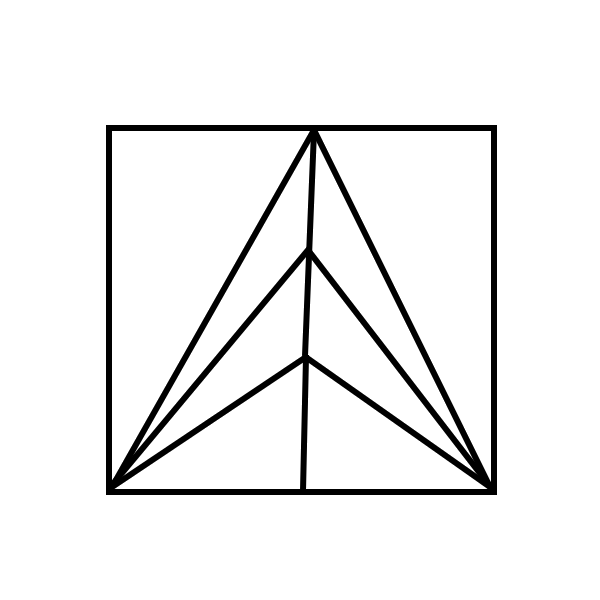Problem 3

Determine which pairs of graphs below are isomorphic.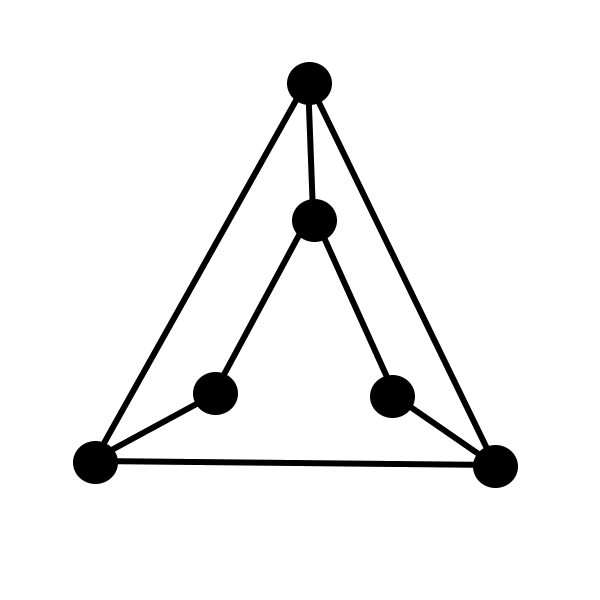$$\quad$$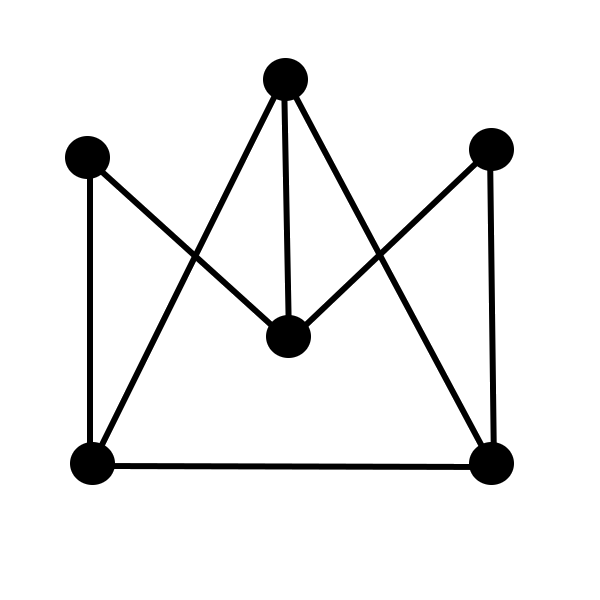$$\quad$$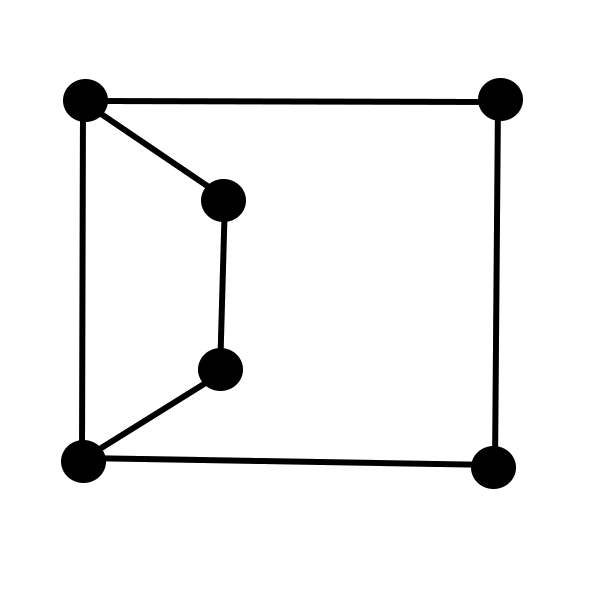Problem 4

Provide example of two relations on the set $G=\{{a},{b},{c},{d}\}$ such that one of them is transitive and the other is not transitive.

Problem 5

The sequence $$(R_n)_{n=0}^{\infty}$$ satisfies $$R_1=6$$ and $R_{n+1}=9R_n+24,\quad\mbox{for }n\geq 1.$ Use the principle of mathematical induction to prove that $$R_n=9^n-3$$ for every integer $$n\geq1$$.

Problem 6

Does there exist a simple graph with $$42$$ vertices $$V_1$$, $$V_2$$, $$V_3$$, $$\dots$$, $$V_{42}$$ such that the degree of each of the vertices $$V_6$$, $$V_7$$, $$\dots$$, $$V_{42}$$ is $$1$$, while the degrees of $$V_1$$, $$V_2$$, $$V_3$$, $$V_4$$, and $$V_5$$ are $$21$$, $$12$$, $$6$$, $$5$$, and $$8$$, respectively?

Problem 7

Prove that for each integer $$k\geq 2$$ there exists a triangle-free simple graph $$G$$ that is not bipartite and whose each vertex has degree $$k$$.